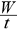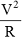Ex﻿

# 1. Electricity

#### Electrical Power

Electrical Power : The rate of loss of energy in an electrical circuit is called electrical power. It is denoted by'P'
p == I2 R = IV =units of power = joule/sec, watt, horse power
1 watt = 1 joule/sec, 1 HP = 746 watt
unit of electrical energy = watt second, kilowatt hour
1 kilowatt hour (kwh) = 3.6 x 106 Joule

If you want to give information about online courses to other students, then share it with more and more on Facebook, Twitter, Google Plus. The more the shares will be, the more students will benefit. The share buttons are given below for your convenience.
×

#### NTSE Physics (Class X)

• Electricity
• Magnetic Effect of Curent
• Light Reflection
• Light Refraction
• Human Eye & Colourful World
• Source of Energy
• Motion

#### NTSE Physics (Class IX)

• Force & laws of motion
• Work and Energy
• Gravitation
• Sound

SHOW CHAPTERS